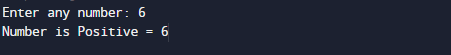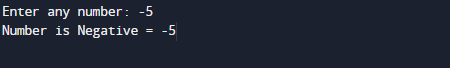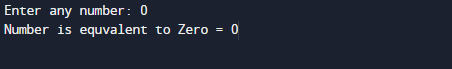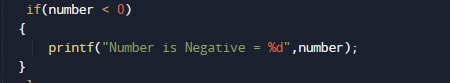C program to check whether a number is positive, negative or zero

In this tutorial you will learn about the C program to check whether a number is positive, negative or zero and its application with practical example.

In this tutorial, we will learn to find whether a number is positive, negative or zero using c programming.

In this tutorial, we will learn to create a C program that will find weather a number is positive, negative or Zero  using C programming.

Prerequisites

Before starting with this tutorial we assume that you are best aware of the following C programming topics:

• C Operators
• If-else statement.
• Nested else-if
• Basic c programming.
• Basic input/output.

C program to check whether a number is positive, negative or zero.

In this program we will find whether a number is positive, negative or zero series using if else statement. We would first declared and initialized the required variables. Next, we would prompt user to input any number. Later we will find that number is positive,negative or zero.

Output

Positive Value.Negative ValueZero Value.In the above program, we have first declared and initialized a set variables required in the program.

• number = it will hold the value of number.

In the next statement user will be prompt to enter any number terms which will be assigned to variable ‘number’.For finding given integer is positive ,negative or zero we will use We will use nested if else statementin above logic inside if we check  if(number > 0), number is greater than zero then number is positive.if(number < 0) if number less than zero then number is negative. and ifif(number == 0), then number is zero.

Here the C program to find whether a number is positive, negative or zero.The above approach is easiest way find the problem. First we check condition for positive number, then for negative or and later we find for zero.

In this tutorial we have learn about the C program to check whether a number is positive, negative or zero and its application with practical example. I hope you will like this tutorial.